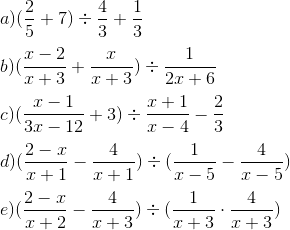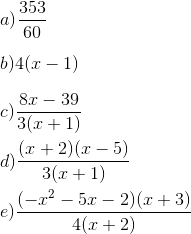# Simplify Rational Expressions? Examples With Detailed Solutions

How to simplify rational expressions? Examples are presented along with detailed solutions and full explanations. More questions with answers at the bottom of the page are also included.

• ## How to simplify rational expressions?

How to simplify rational expressions? The rules of addition, subtraction, multiplication and division of rational expressions are used to simplify complex expressions.
Rational expressions with the same denominator are added or subtracted as follows:Rational expressions are multiplied as follows: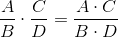We divide two rational expressions by multiplying the first rational expression by the reciprocal of the second rational expression as follows:If you have difficulties in simplifying rational expressions, review the tutorials in How to Add, Subtract and Simplify Rational Expressions and How to multiply, divide and simplify rational expressions and then start the present tutorial. The examples with detailed solutions and explanations in this tutorials will help you overcome any difficulties in simplifying rational expressions on the condition that you understand every step involved in solving these questions and also spend more time to practice if needed. I will present the examples below starting with fractions first and then with rational expressions, with more challenging questions as you walk through the tutorial. You need to understand each step!

## Example 1

Simplify:.
Solution:
We first convert to a common denominator using the LCD (least common denominator) of 3 and 2 which is 6.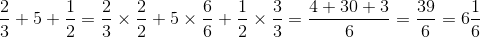## Example 2

Simplify:.
Solution:
We simplify by converting to the same denominator using the LCD of (x - 2) and (x + 1) which is is (x - 2)(x + 1) and applying the rule of the sum of two rational expressions.## Example 3

Simplify:.
Solution:
We simplify by converting to the same denominator## Example 4

Simplify:Solution:
Apply the rule of subtraction of rational expressions to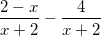and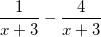then simplify.Apply the rule of division of rational expressions ( which becomes a multiplication by the reciprocal ) and factorand simplify## Example 5

Simplify:Solution:
Apply the rule of multiplication toand the rule of subtraction to.Apply the rule of division (multiply be the reciprocal):Simplify.## More Questions: Simplify the following expressions Answers at the bottom of the page